【BP预测】基于灰狼算法改进BP神经网络实现数据预测

一、 BP神经网络预测算法简介

1.1 受相关指标影响的BP神经网络算法原理

1）输入层：相当于人的五官，五官获取外部信息，对应神经网络模型input端口接收输入数据的过程。
2）隐含层：对应人的大脑，大脑对五官传递来的数据进行分析和思考，神经网络的隐含层hidden Layer对输入层传来的数据x进行映射，简单理解为一个公式hiddenLayer_output=F(w*x+b)。其中，w、b叫做权重、阈值参数，F()为映射规则，也叫激活函数，hiddenLayer_output是隐含层对于传来的数据映射的输出值。换句话说，隐含层对于输入的影响因素数据x进行了映射，产生了映射值。
3）输出层：可以对应为人的四肢，大脑对五官传来的信息经过思考（隐含层映射）之后，再控制四肢执行动作（向外部作出响应）。类似地，BP神经网络的输出层对hiddenLayer_output再次进行映射，outputLayer_output=w *hiddenLayer_output+b。其中，w、b为权重、阈值参数，outputLayer_output是神经网络输出层的输出值（也叫仿真值、预测值）（理解为，人脑对外的执行动作，比如婴儿拍打桌子）。
4）梯度下降算法：通过计算outputLayer_output和神经网络模型传入的y值之间的偏差，使用算法来相应调整权重和阈值等参数。这个过程，可以理解为婴儿拍打桌子，打偏了，根据偏离的距离远近，来调整身体使得再次挥动的胳膊不断靠近桌子，最终打中。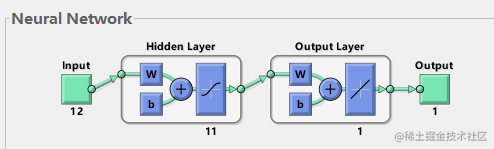二、灰狼算法

2 算法原理：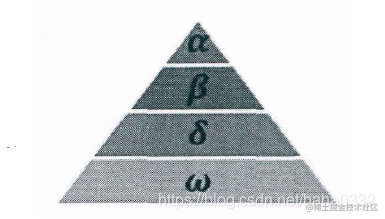**社会等级第一层：**狼群中的头狼记为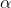​，​ 狼主要负责对捕食、栖息、作息时间等活动作出决策。由于其它的狼需要服从​ 狼的命令，所以​ 狼也被称为支配狼。另外，​ 狼不一定是狼群中最强的狼，但就管理能力方面来说，​ 狼一定是最好的。

GWO 优化过程包含了灰狼的社会等级分层跟踪包围攻击猎物等步骤，其步骤具体情况如下所示。

１）社会等级分层（Social Hierarchy）当设计 GWO 时，首先需构建灰狼社会等级层次模型。计算种群每个个体的适应度，将狼群中适应度最好的三匹灰狼依次标记为​、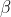​ 、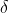​ ，而剩下的灰狼标记为​。也就是说，灰狼群体中的社会等级从高往低排列依次为；​、​ 、​ 及​。GWO 的优化过程主要由每代种群中的最好三个解（即​、​ 、​ ）来指导完成。

２）包围猎物（ Encircling Prey ）灰狼捜索猎物时会逐渐地接近猎物并包围它，该行为的数学模型如下：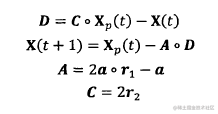３）狩猎（ Hunring）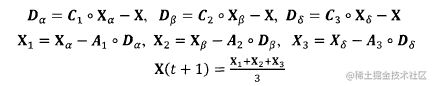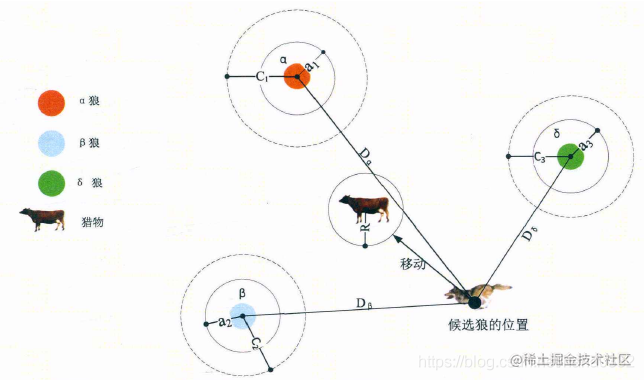４）攻击猎物（Attacking Prey）构建攻击猎物模型的过程中，根据2)中的公式，ａ值的减少会引起 A 的值也随之波动。换句话说，A 是一个在区间［-a，a］**（备注：原作者的第一篇论文里这里是[-2a,2a]，后面论文里纠正为[-a,a]）**上的随机向量，其中ａ在迭代过程中呈线性下降。当 A 在［-1，１］区间上时，则捜索代理（Search Agent）的下一时刻位置可以在当前灰狼与猎物之间的任何位置上。

５）寻找猎物（Search for Prey）灰狼主要依赖​、​ 、​ 的信息来寻找猎物。它们开始分散地去搜索猎物位置信息，然后集中起来攻击猎物。对于分散模型的建立，通过｜A｜＞１使其捜索代理远离猎物，这种搜索方式使 GWO 能进行全局搜索。GWO 算法中的另一个搜索系数是Ｃ。从2)中的公式可知，Ｃ向量是在区间范围［０，２］上的随机值构成的向量，此系数为猎物提供了随机权重，以便増加（｜Ｃ｜＞１）或减少（｜Ｃ｜＜１）。这有助于 GWO 在优化过程中展示出随机搜索行为，以避免算法陷入局部最优。值得注意的是，Ｃ并不是线性下降的，Ｃ在迭代过程中是随机值，该系数有利于算法跳出局部，特别是算法在迭代的后期显得尤为重要。

三、部分代码

% Grey Wolf Optimizer
function [Alpha_score,Alpha_pos,Convergence_curve]=GWO(SearchAgents_no,Max_iter,lb,ub,dim,fobj)

% initialize alpha, beta, and delta_pos
Alpha_pos=zeros(1,dim);
Alpha_score=inf; %change this to -inf for maximization problems

Beta_pos=zeros(1,dim);
Beta_score=inf; %change this to -inf for maximization problems

Delta_pos=zeros(1,dim);
Delta_score=inf; %change this to -inf for maximization problems

%Initialize the positions of search agents
Positions=initialization(SearchAgents_no,dim,ub,lb);

%%%%%%%%%%%%%%%%%%%%%%%%%%%%%%%%%%%%%TRANSFORM HERE BY EQ1%%%%%%%%%%%%%%%%%%%%%%%%%%%%%%%%%%%%%%%%%%%%%%%%%%
Convergence_curve=zeros(1,Max_iter);

l=0;% Loop counter

%%%%%%%%%%%%%%%%%%%%%%%%%%%%EVALUAGE J HERE F?RST FOR ALL X? EQ2%%%%%%%%%%%%%%%%%%%%%%%%%%%%%%%%%%%%%%%%%

% Main loop STEP 3
while l<Max_iter
for i=1:size(Positions,1)

% Return back the search agents that go beyond the boundaries of the search space
Flag4ub=Positions(i,:)>ub;
Flag4lb=Positions(i,:)<lb;
Positions(i,:)=(Positions(i,:).*(~(Flag4ub+Flag4lb)))+ub.*Flag4ub+lb.*Flag4lb;

% Calculate objective function for each search agent
fitness=fobj(Positions(i,:));

% Update Alpha, Beta, and Delta
if fitness<Alpha_score
Alpha_score=fitness; % Update alpha
Alpha_pos=Positions(i,:);
end

if fitness>Alpha_score && fitness<Beta_score
Beta_score=fitness; % Update beta
Beta_pos=Positions(i,:);
end

if fitness>Alpha_score && fitness>Beta_score && fitness<Delta_score
Delta_score=fitness; % Update delta
Delta_pos=Positions(i,:);
end
end

a=2-l*((2)/Max_iter); % a decreases linearly fron 2 to 0

% Update the Position of search agents including omegas
for i=1:size(Positions,1)
for j=1:size(Positions,2)

r1=rand(); % r1 is a random number in [0,1]
r2=rand(); % r2 is a random number in [0,1]

A1=2*a*r1-a; % Equation (3.3)
C1=2*r2; % Equation (3.4)

D_alpha=abs(C1*Alpha_pos(j)-Positions(i,j)); % Equation (3.5)-part 1
X1=Alpha_pos(j)-A1*D_alpha; % Equation (3.6)-part 1

r1=rand();
r2=rand();

A2=2*a*r1-a; % Equation (3.3)
C2=2*r2; % Equation (3.4)

D_beta=abs(C2*Beta_pos(j)-Positions(i,j)); % Equation (3.5)-part 2
X2=Beta_pos(j)-A2*D_beta; % Equation (3.6)-part 2

r1=rand();
r2=rand();

A3=2*a*r1-a; % Equation (3.3)
C3=2*r2; % Equation (3.4)

D_delta=abs(C3*Delta_pos(j)-Positions(i,j)); % Equation (3.5)-part 3
X3=Delta_pos(j)-A3*D_delta; % Equation (3.5)-part 3

Positions(i,j)=(X1+X2+X3)/3;% Equation (3.7)

end
end
%%%%%%%%%%%%%%%%%%%%%%%%%%%%EVALUAGE J HERE F?RST FOR ALL X? EQ2%%%%%%%%%%%%%%%%%%%%%%%%%%%%%%%%%
l=l+1;
Convergence_curve(l)=Alpha_score;
end四、仿真结果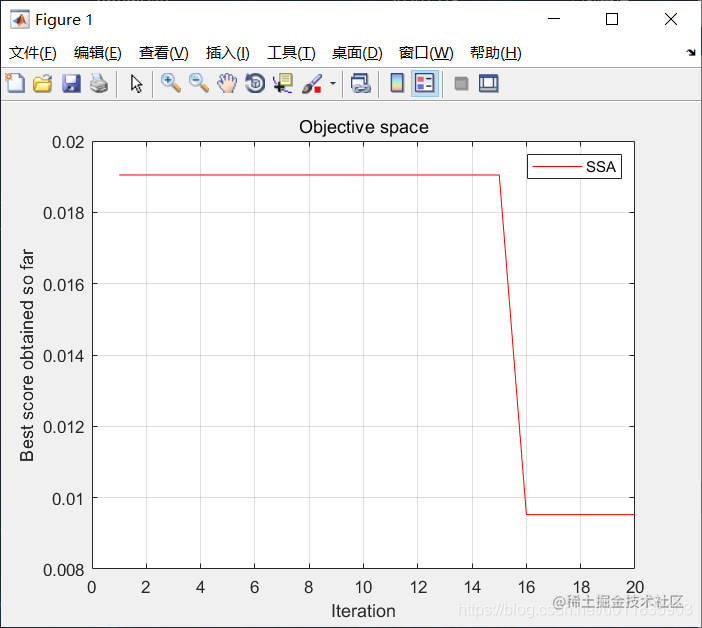BP神经网络

100%

95%

GWO-BP

100%

99.8%

五、参考文献及代码私信博主

《基于BP神经网络的宁夏水资源需求量预测》